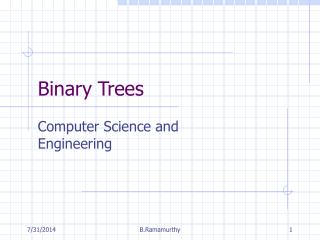DownloadDownload PresentationBinary Trees

# Binary Trees

Télécharger la présentation## Binary Trees

- - - - - - - - - - - - - - - - - - - - - - - - - - - E N D - - - - - - - - - - - - - - - - - - - - - - - - - - -
##### Presentation Transcript

1. Binary Trees Computer Science and Engineering B.Ramamurthy

2. Introduction • We studied linked list is a dynamic linear data structure. • It is dynamic since it supports efficient addition and deletion of items. • It is linear since it is sequential and each element in it has exactly one successor. • A tree is nonlinear data structure. • Each element may have more than one successor. • Can be static or dynamic. B.Ramamurthy

3. Topics for Discussion • Elements of a tree • Examples of trees • Binary Tree Definition • Types of binary trees • Contiguous (static) representation • Dynamic representation B.Ramamurthy

4. Terminology • Trees are used to represent relationships: items in a tree are referred to as nodes and the lines connecting the nodes that express the hierarchical relationship are referred to as edges. • The edges in a tree are directed. • Trees are hierarchical which means that a parent-child relationship exist between the nodes in the tree. • Each node has at most one parent. The node with no parents is the root node. • The nodes that have no successors (no children nodes) are known as leaf nodes. • Lets look at some examples and identify the various elements. B. Ramamurthy

5. Examples • Family ancestor tree • Directory of files organization in your computer system • Parse tree • Languages are defined using grammar • Grammars are specified using rules or syntax • Syntax is expressed using a notation called Backaus-Naur Form (BNF) (John Backus and Peter Naur) • Expression trees • Game trees B.Ramamurthy

6. An Ancester Tree (From Greek mythology) Gaea Cronus Phoebe Ocean Zeus Poseidon Demeter Pluto Leto ……… Apollo B.Ramamurthy

7. BNF for a Language • BNF notation includes nonterminals and terminals. • Terminals are literals or particular symbols. • Nonterminals are general expressions that can be substituted with terminals and nonterminals. Grammar rules specify the definition of a nonterminal. Nonterminals are enclosed with angle brackets <nonterminal> • Symbols used in construction include ::= (defines), | (or) and other common operators. B.Ramamurthy

8. BNF for a Java Statement <statement> ::= <selection-stmt> | <other-stmt> <selection-stmt> ::= if (<expr>) <statement> else <statement> <expr>::= <relational-expr>|<assign-expr>|<identifier> <relational-expr> ::= <expr> <rel-op> <expr> <assign-expr> ::= <expr> = <expr> B.Ramamurthy

9. Parse tree <statement> <selection-stmt> if ( <expr> ) <statement> else <statement> … <expr> <relational-expr> <expr> <expr> <rel-op> …. A major task of the compiler is to construct a parse tree from the input program and verify it is correct. <identifier> <identifier> < b a B.Ramamurthy

10. Expression tree + A + B + C * D + <left><root><right> (in-order expression) <root><left><right> (pre-order expression> <left><right><root> (post-order expression) * A B C D Single representation; Multiple views B.Ramamurthy

11. X X X X X X X X X X X X Game Tree …… X X X …. … X X X X X X B.Ramamurthy

12. Binary Tree • A binary tree can be defined recursively as follows. It is either • empty, or • consists of a root node together with left and right trees, both of which are binary trees. B.Ramamurthy

13. Binary Tree NonEmpty Empty NullObject (pattern) Singleton (pattern) B.Ramamurthy

14. Binary Tree (contd.) B.Ramamurthy

15. Binary Tree (contd.) B.Ramamurthy

16. Characteristics of trees • A path is a sequence of nodes n1, n2, ..., nk such that node ni is the parent of node ni+1 for all 1 <= i <= k. • The length of a path is the number of edges on the path. • The height of a node is the length of the longest path from the node to a leaf. • The height of tree is the height of its root. • The level of a node is the length of the path from the root to the node. B.Ramamurthy

17. Full Binary Tree • A full binary tree is a tree in which each node has exactly zero or two non-empty children. All leaves are at the same level. • A complete binary tree in which all the leaf nodes are in level n or n-1 and all leaves on the level n are filled from left to right. • There are some interesting properties that arise out of this definition. • Lets look at some examples to illustrate the various definitions. B.Ramamurthy

18. Example root Level 0 Level 1 internal node Height of the tree:3 leaf B.Ramamurthy